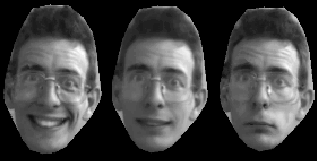# Combined Appearance Models

We can model the shape change of an object using a Statistical Shape Model.. We can use a similar statistical model to represent the intensity variation across a region. Given a set of training images, labelled with landmark points, we can use image warping to deform each image so that the object has the mean shape, then build a statistical model of the grey-levels across the object.

We can then use the shape and grey-level parameters to synthise new examples. Given a set of grey-level parameters, we generate the grey-level image with a mean shape. We then warp that image to match the shape defined by the shape parameters.

Of course, in many cases the shape and grey-level parameters will be correllated, and we can build a model to represent this. For instance, we have built a model of the appearance of my face. Here is one of the modes of combined shape and grey-level appearance:(The central image is the mean)

Such models can be fit to new images using the Active Appearance Model algorithm.

Tim Cootes